# 2D Integrals : Gaussian Quadrilateral Example

## A first example on the reference quadrilateral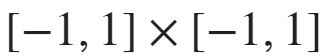Let's consider the function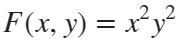defined on the refrence quadrilateral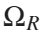defined with vertices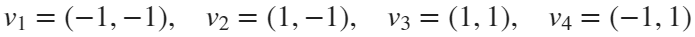.
We want to compute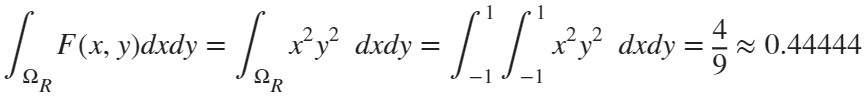The 2D Gauss formula is similar to the 1D case but using the 2D Gauss points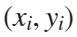with weights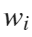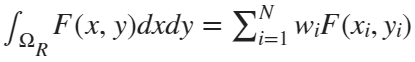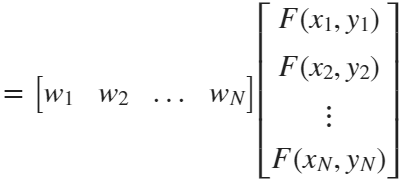. where N is the total number of Gauss points.

### Compute the 2D Gauss points on the reference element

First we compute the appropriate Gauss points in the reference quadrilateral. The Gauss points in the reference quadrilateral are obtained from the 1D Gauss points in [-1,1] in both axes x and y and are obtained using the Matlab function gaussValues2DQuad found at the end of this script.
We can use a Gauss quadrature using only Nax=2 (in each axis) in this example, because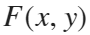is a polynomial function of degree less than 3 in each variable.
Nax=2; %order of the Gaussian quadrature
fprintf('N = %d, w= %f %f %f %f\n', 2*Nax,w);
N = 4, w= 1.000000 1.000000 1.000000 1.000000
ptGaussRef % +/- 1/sqrt(3)
ptGaussRef = 4×2
-0.5774 -0.5774 -0.5774 0.5774 0.5774 -0.5774 0.5774 0.5774
% Draw Gauss points in the reference quadrilateral [-1,1]x[-1,1]
vertices=[-1 -1; 1,-1; 1,1; -1,1; -1 -1];
plot(vertices(:,1), vertices(:,2));
hold on;
plot(ptGaussRef(:,1),ptGaussRef(:,2),'ro');
hold off;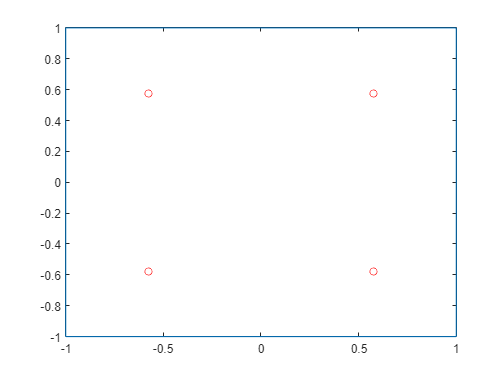F=@(x,y) (x.^2).*y.^2;
Fxy=F(ptGaussRef(:,1),ptGaussRef(:,2));
Integ=w*Fxy
Integ = 0.4444

## General case: Define the function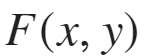and the domain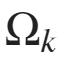Let's consider the function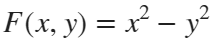defined on the quadrilateral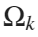with vertices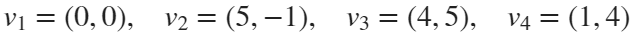.
We want to compute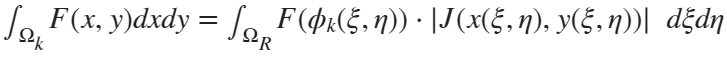,
where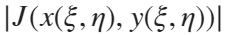is the absolute value of the determinat of the Jacobian of the variable change of coordinates.
Below, you have an slide showing the change of variables needed to relate the reference quadrilateral [-1,1]x[-1,1] with a general one. The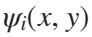are known as shape functions associated to each vertice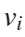and are explained below: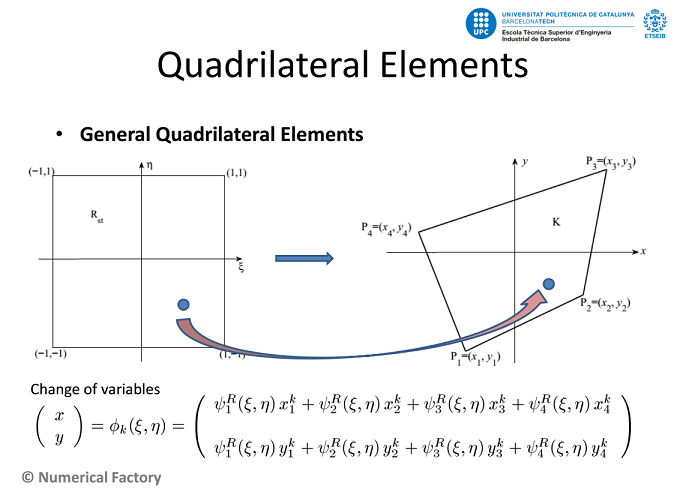## Example:

Now consider our function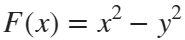and we code in Matlab the above change of variable formulas.

### Compute the change of variables functions

As it is shown in the previous figure, the needed functions to define the change of variablesare known as shape functions. These functions are associated to the respective vertex of the reference quadrilateral and they satisfy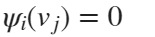if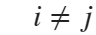and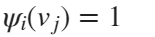if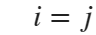The definition of the 4 shape functions associated to each vertex of the reference quadrilateral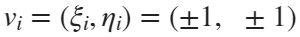are respectively: (check the above properties)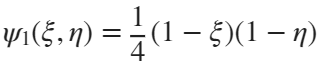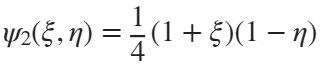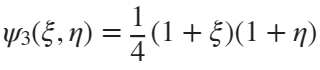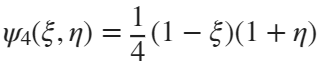% The present function
F =@(x,y) (x.^2)- y.^2;
% The domain vertices
v1=[0,0];
v2=[5,-1];
v3=[4,5];
v4=[1,4];
% We use Matlab *implicit function* definition:
%
% Shape functions
Psi1=@(xi,eta)(1-xi).*(1-eta)/4;
Psi2=@(xi,eta)(1+xi).*(1-eta)/4;
Psi3=@(xi,eta)(1+xi).*(1+eta)/4;
Psi4=@(xi,eta)(1-xi).*(1+eta)/4;
% Shape function derivatives
dPsi11=@(xi,eta) -(1-eta)/4;
dPsi21=@(xi,eta) (1-eta)/4;
dPsi31=@(xi,eta) (1+eta)/4;
dPsi41=@(xi,eta) -(1+eta)/4;
dPsi12=@(xi,eta) -(1-xi)/4;
dPsi22=@(xi,eta) -(1+xi)/4;
dPsi32=@(xi,eta) (1+xi)/4;
dPsi42=@(xi,eta) (1-xi)/4;
Jacb =@(xi,eta) [dPsi11(xi,eta), dPsi21(xi,eta),dPsi31(xi,eta),dPsi41(xi,eta);...
dPsi12(xi,eta), dPsi22(xi,eta),dPsi32(xi,eta),dPsi42(xi,eta)];

### Compute the corresponding Gaussian points on the domain

The change of variables from the reference quadrilateral to a general one is: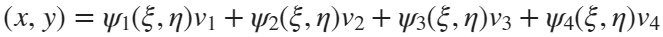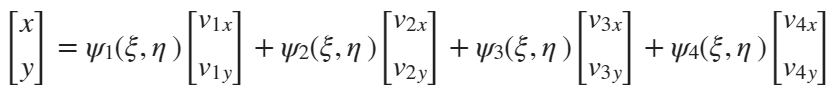evaluate Shape functions on Gaussian reference points
xi = ptGaussRef(:,1);
eta = ptGaussRef(:,2);
evalPsi1 = Psi1(xi,eta);
evalPsi2 = Psi2(xi,eta);
evalPsi3 = Psi3(xi,eta);
evalPsi4 = Psi4(xi,eta);
% change these points to the new quadrilateral(from the change of variables function)
ptGaussDomain = evalPsi1*v1+evalPsi2*v2+evalPsi3*v3+evalPsi4*v4;
% Draw Gauss points in the present domain
figure()
vertices=[v1;v2;v3;v4;v1];
plot(vertices(:,1), vertices(:,2));
hold on;
plot(ptGaussDomain(:,1),ptGaussDomain(:,2),'ro');
hold off;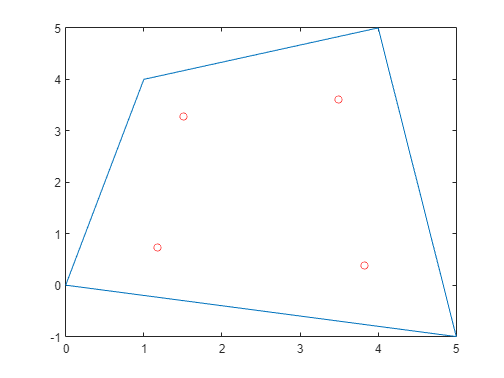### Compute the Jacobian terms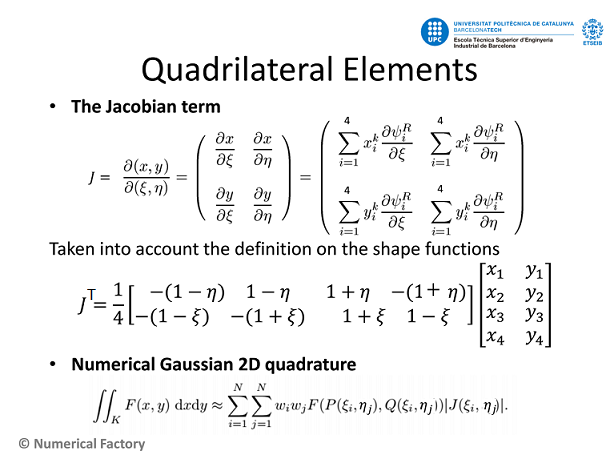vertex matrix
v = [v1;v2;v3;v4];
% evaluate Jacobian contribution for each Gauss point
for i=1:size(xi,1)
evalDetJacb(i) = abs(det(Jacb(xi(i),eta(i))*v));
end

### Compute the integral value according Gauss formula

%evaluate the function on the domain points
evalF=F(ptGaussDomain(:,1),ptGaussDomain(:,2));

% Finally, apply Gauss integration formula
suma=0;
for i=1:size(ptGaussDomain,1)
suma=suma+w(i)*evalF(i)*evalDetJacb(i);
end
integ = suma
integ = 60.0000

## Exercise 1: Build the integQuad function

From the previous example, one can build a function that returns the value of the integral of an inline function defined on a quadrilateral domain (see at the end of this document).
where
• F: is an inline function previously defined
• vertices: are the coordinates of the quadrilateral vertices.
• N: order of Gauss integration
(Hint: find the answer at the end of this document)

## Application: Integration over a mesh

Let's consider the integral of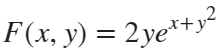in a general rectangular domain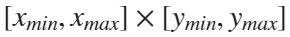, in particular we know that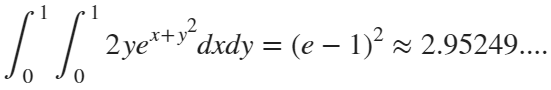Instead of integrating only in one quadrilateral, we want to show how to approximate the integral value using a mesh subdivision of the domain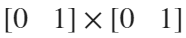The final integral will be the sum of the integrals in each small quadrilateral. Therefore, we increase the precission on the final value.
F=@(x,y) 2*exp(x+y.^2).*y; %present function
xmin=0; % rectangle dimensions
xmax=1;
ymin=0;
ymax=1;

% -----------------------------------------------
% In order to create a rectangular mesh in Matlab
% we can make the following procedure
% -----------------------------------------------

numDiv=4; %number of subdivisions
hx=(xmax-xmin)/numDiv;
hy=(ymax-ymin)/numDiv;
xi=xmin:hx:xmax; %all points in the x-axis
eta=ymin:hy:ymax; %all points in the y-axis
[X,Y] = meshgrid(xi,eta); % create a grid of points
Z = F(X,Y); % function values
surf(X,Y,Z); % optional: is just to visualize the result
title('Plot of F(x,y) in the mesh domain')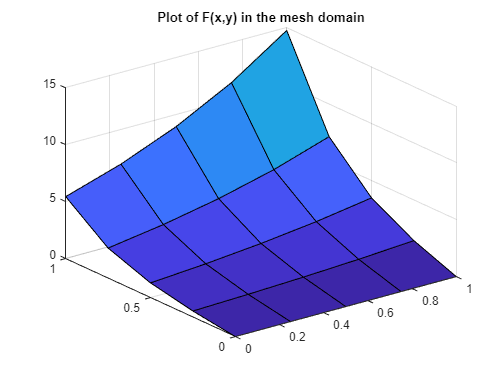Hint. Here we plot the surface as the approximation of the continous function using the mesh
[elem,vertex] = surf2patch(X,Y,Z); % the main variables
elem = 16×4
1 6 7 2 2 7 8 3 3 8 9 4 4 9 10 5 6 11 12 7 7 12 13 8 8 13 14 9 9 14 15 10 11 16 17 12 12 17 18 13
vertex = 25×3
0 0 0 0 0.2500 0.5322 0 0.5000 1.2840 0 0.7500 2.6326 0 1.0000 5.4366 0.2500 0 0 0.2500 0.2500 0.6834 0.2500 0.5000 1.6487 0.2500 0.7500 3.3803 0.2500 1.0000 6.9807
numElem=size(elem,1); %total number of elements
numVert= size(vertex,1); % total number of vertices
fprintf('num Elements =%d, num vertices = %d \n',numElem,numVert)
num Elements =16, num vertices = 25

##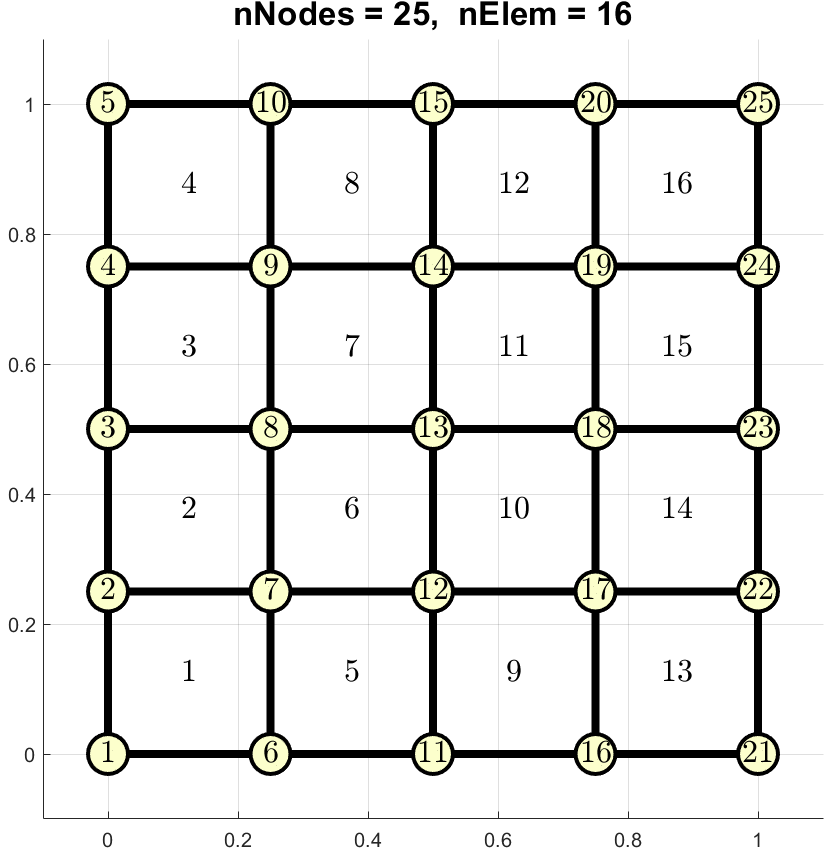Fig. Visualization of the numbering for vertex and elements for a 4x4 mesh.

### Structure of the Mesh

In general a mesh is defined by two tables: vertex and elem.
vertex: is a matrix with 3 columns and numVert rows, where numVert is the number of grid points.
For each row:
• the two first coordinates are the (x,y) grid values
• the third coordinate is the function value: z=F(x,y)
elem: is a numElem×4 matrix, where numElem is the number of elements For each row:
• the four numbers are the number of the vertices defining this element
N=2; %number of Gauss points = NxN
integralTot=0;
for i=1:numElem %compute the integral on each element
n1=elem(i,1); %first vertex of the i-th element
v1=[vertex(n1,1),vertex(n1,2)];
n2=elem(i,2); %second vertex of the i-th element
v2=[vertex(n2,1),vertex(n2,2)];
n3=elem(i,3); %third vertex of the i-th element
v3=[vertex(n3,1),vertex(n3,2)];
n4=elem(i,4); %fourth vertex of the i-th element
v4=[vertex(n4,1),vertex(n4,2)];
vertices=[v1;v2;v3;v4];
integralTot=integralTot+elemInteg;
end
actualIntegVal= (exp(1)-1)^2 %exact known value
actualIntegVal = 2.9525
errorInt=abs(actualIntegVal-integralTot) %absolute error
errorInt = 2.9420e-04

### Gauss points in 2D

They are the same as in the 1D version, but now using the points in both x and y axis.
switch (n)
case 1
w=2; pt=0;
case 2
w=[1,1]; pt=[-1/sqrt(3), 1/sqrt(3)];
case 3
w=[5/9, 8/9, 5/9]; pt=[-sqrt(3/5), 0, sqrt(3/5)];
case 4
w=[(18+sqrt(30))/36, (18+sqrt(30))/36, (18-sqrt(30))/36, (18-sqrt(30))/36];
pt=[-sqrt(3/7-2/7*(sqrt(6/5))), sqrt(3/7-2/7*(sqrt(6/5))),...
-sqrt(3/7+2/7*(sqrt(6/5))), sqrt(3/7+2/7*(sqrt(6/5)))];
case 5
w=[(322+13*sqrt(70))/900, (322+13*sqrt(70))/900, 128/225,...
(322-13*sqrt(70))/900,(322-13*sqrt(70))/900];
pt=[-1/3*sqrt(5-2*sqrt(10/7)), 1/3*sqrt(5-2*sqrt(10/7)),0,...
-1/3*sqrt(5+2*sqrt(10/7)), 1/3*sqrt(5+2*sqrt(10/7))];
otherwise
error('No data are defined for this value');
end
pt2D=[];
w2D=[];
for i=1:n
for j=1:n
pt2D=[pt2D; [pt(i),pt(j)]];
w2D=[w2D,w(i)*w(j)];
end
end
end

Copy this function in a new file named integQuad.m
% Shape functions
Psi1=@(x,y)(1-x).*(1-y)/4;
Psi2=@(x,y)(1+x).*(1-y)/4;
Psi3=@(x,y)(1+x).*(1+y)/4;
Psi4=@(x,y)(1-x).*(1+y)/4;
% Shape function derivatives
dPsi11=@(x,y) -(1-y)/4;
dPsi21=@(x,y) (1-y)/4;
dPsi31=@(x,y) (1+y)/4;
dPsi41=@(x,y) -(1+y)/4;
dPsi12=@(x,y) -(1-x)/4;
dPsi22=@(x,y) -(1+x)/4;
dPsi32=@(x,y) (1+x)/4;
dPsi42=@(x,y) (1-x)/4;
Jacb =@(x,y) [dPsi11(x,y), dPsi21(x,y),dPsi31(x,y),dPsi41(x,y);...
dPsi12(x,y), dPsi22(x,y),dPsi32(x,y),dPsi42(x,y)];
% evaluate Shape functions on Gaussian reference points
xi = ptGaussRef(:,1);
eta = ptGaussRef(:,2);
evalPsi1 = Psi1(xi,eta);
evalPsi2 = Psi2(xi,eta);
evalPsi3 = Psi3(xi,eta);
evalPsi4 = Psi4(xi,eta);
% from the change of variables function
ptGaussDomain = [evalPsi1,evalPsi2,evalPsi3,evalPsi4]*vertices;
% evaluate Jacobian contribution for each point
for i=1:size(xi,1)
evalDetJacb(i) = abs(det(Jacb(xi(i),eta(i))*vertices));
end
%evaluate the function on the domain points
evalF=F(ptGaussDomain(:,1),ptGaussDomain(:,2));
% Finally, apply Gauss formula
suma=0;
for i=1:size(ptGaussDomain,1)
suma=suma+w(i)*evalF(i)*evalDetJacb(i);
end
valInteg = suma;
end

## Exercise 2:

Increase the number of subdivisions and see how the error evolves.
(c) Numerical Factory 2022